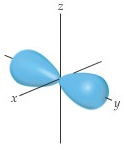# Problem: Classify the following statements as either true or false:In a contour representation of an orbital, such as the one shown here for a 2p orbital, the electron is confined to move about the nucleus on the outer surface of the shape.

###### FREE Expert Solution

We're asked to classify the following statement as either true or false:

In a contour representation of an orbital, such as the one shown here for a 2p orbital, the electron is confined to move about the nucleus on the outer surface of the shape.

Recall that orbitals describe where an electron is most likely to be found

For the given contour representation of the 2p orbital, the regions within the blue shade describe where the electrons are most likely to be found###### Problem Details

Classify the following statements as either true or false:

In a contour representation of an orbital, such as the one shown here for a 2p orbital, the electron is confined to move about the nucleus on the outer surface of the shape.